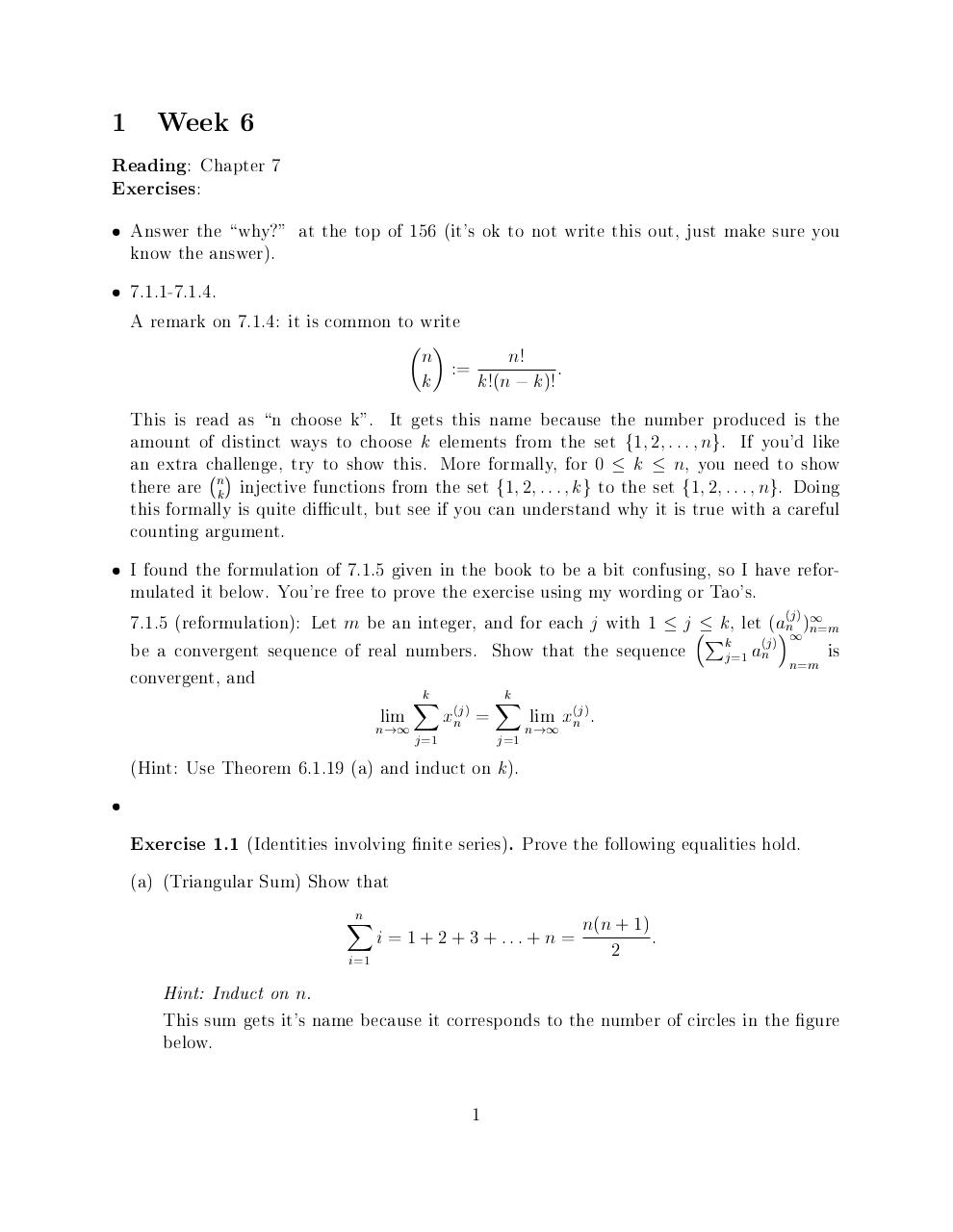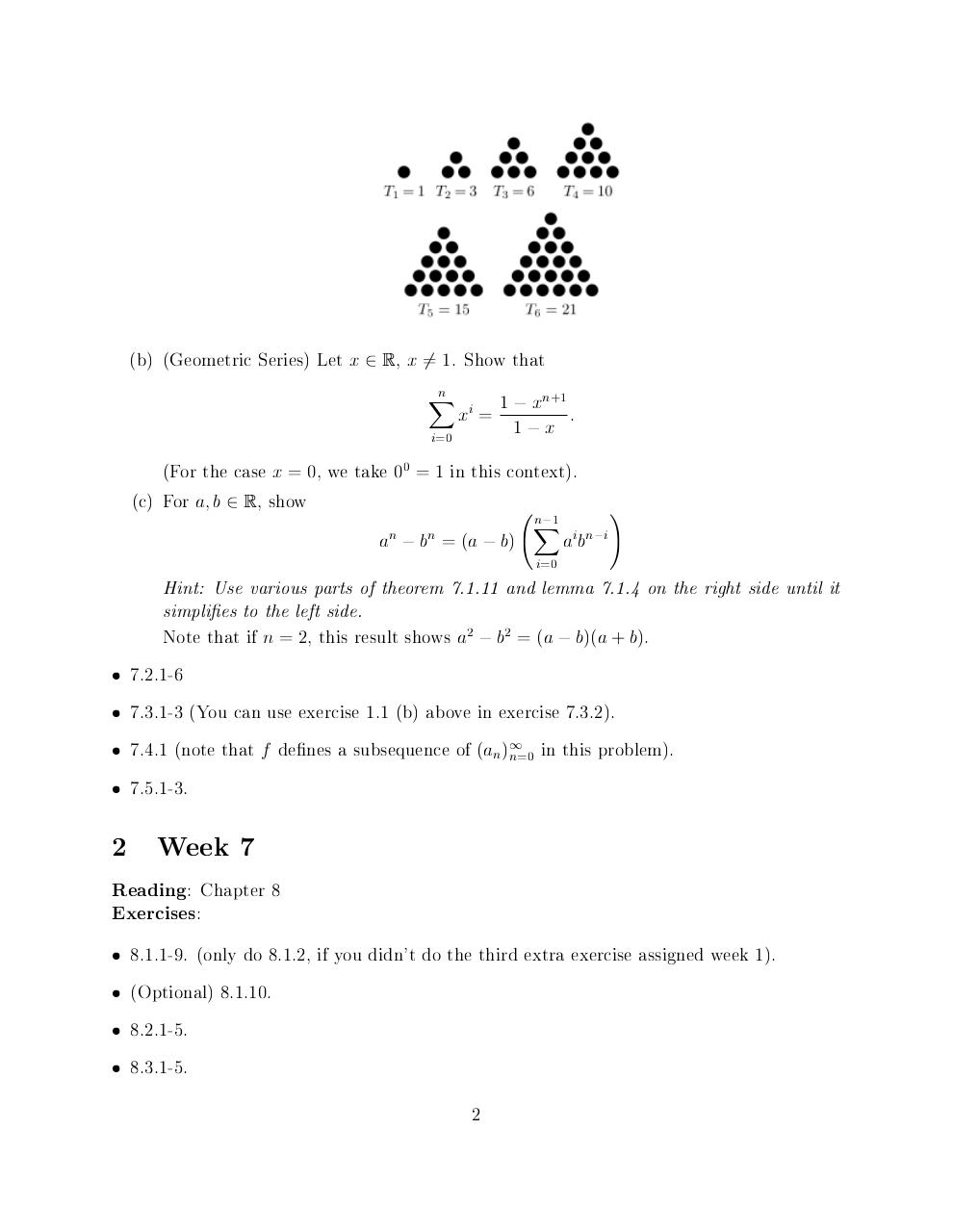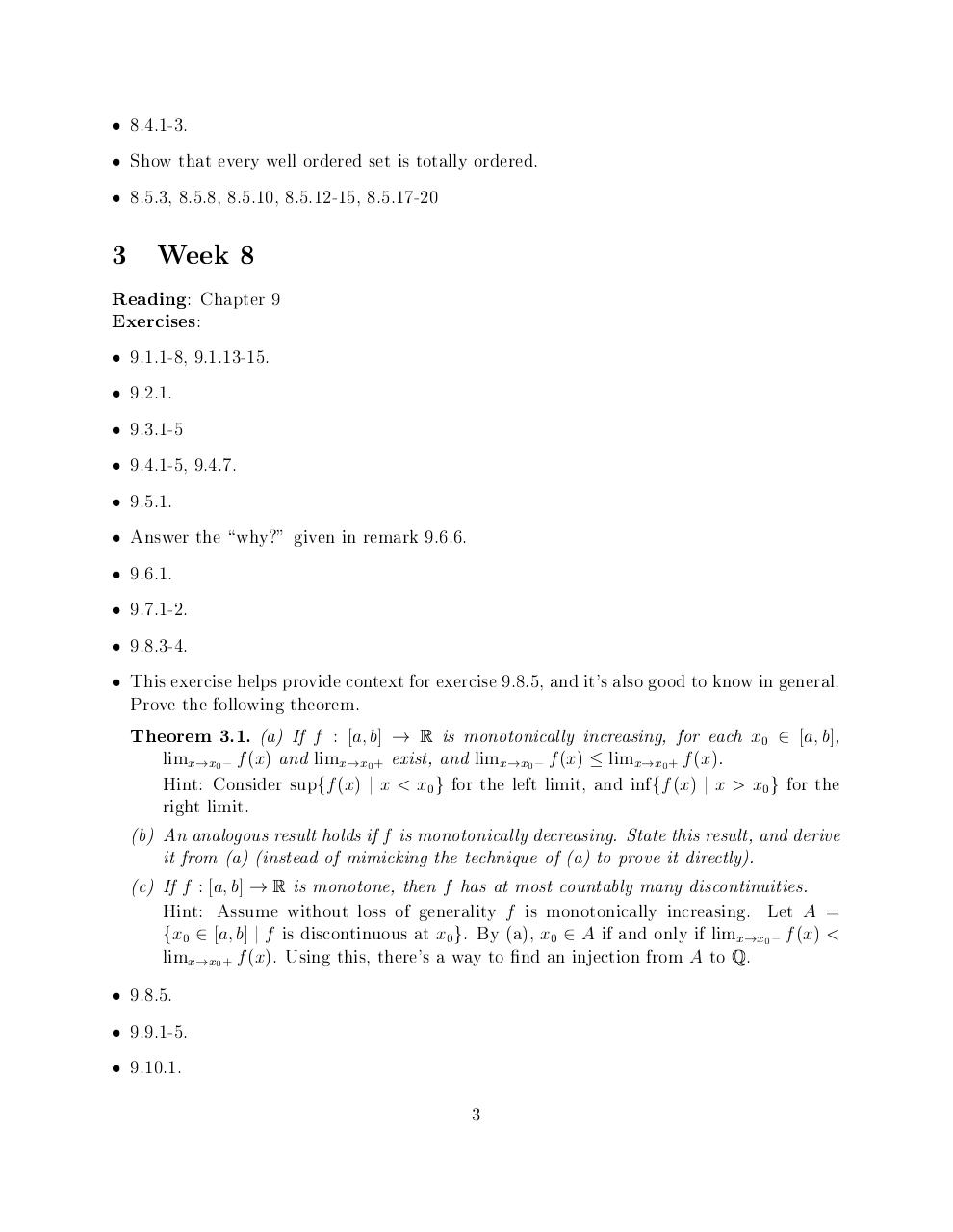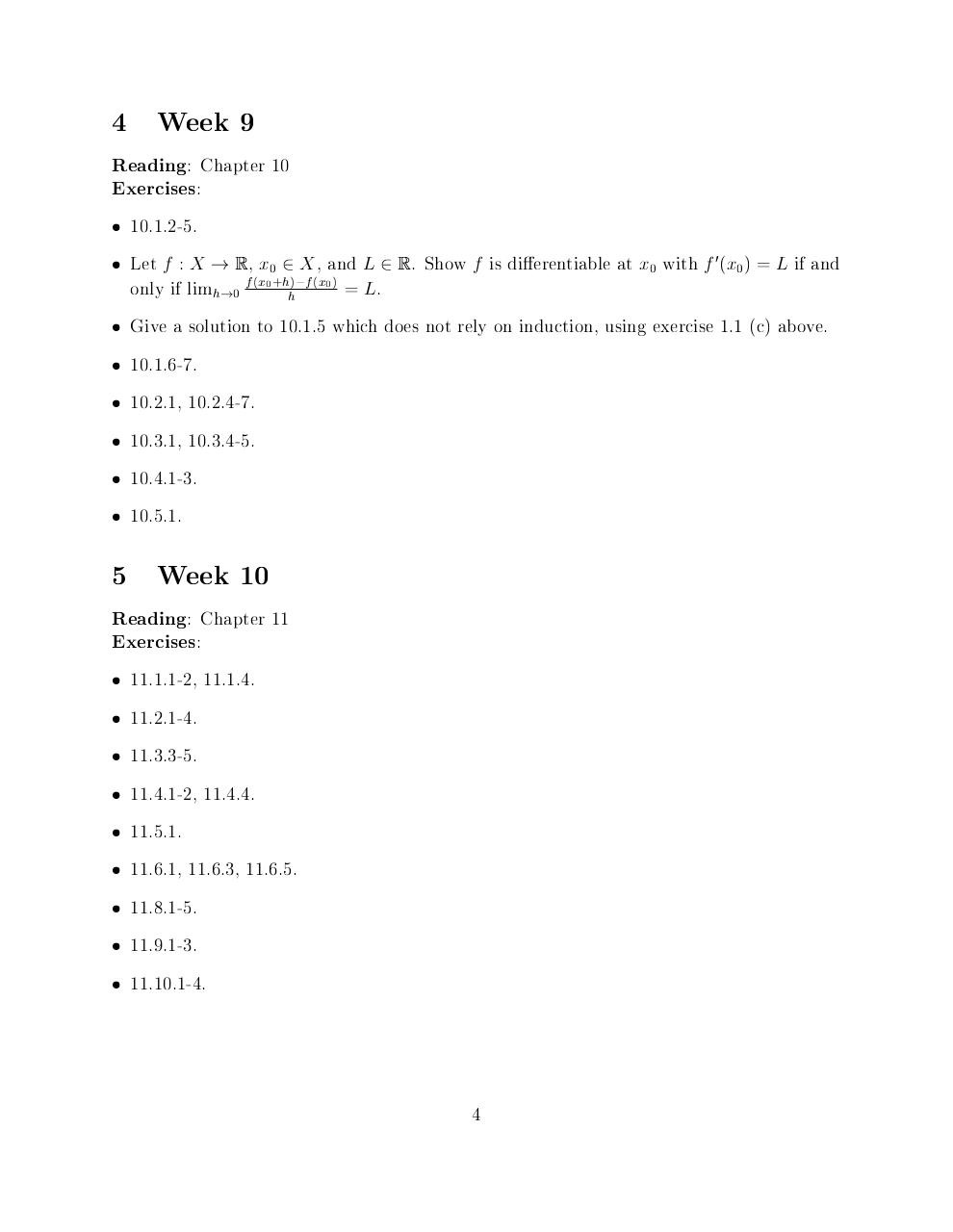# assignments .pdf

### File information

Original filename: assignments.pdf

This PDF 1.5 document has been generated by TeX / pdfTeX-1.40.17, and has been sent on pdf-archive.com on 24/06/2017 at 05:02, from IP address 74.66.x.x. The current document download page has been viewed 234 times.
File size: 75 KB (4 pages).
Privacy: public file

assignments.pdf (PDF, 75 KB)

### Document preview

1

Week 6

: Chapter 7
Exercises:



Answer the \why?" at the top of 156 (it's ok to not write this out, just make sure you



7.1.1-7.1.4.
A remark on 7.1.4: it is common to write
 
n
k

:=

!

n
k

!(n

k

)!

:

This is read as \n choose k". It gets this name because the number produced is the
amount of distinct ways to choose k elements from the set f1; 2; : : : ; ng. If you'd like
an extra challenge,
try to show this. More formally, for 0  k  n, you need to show

n
there are k injective functions from the set f1; 2; : : : ; kg to the set f1; 2; : : : ; ng. Doing
this formally is quite dicult, but see if you can understand why it is true with a careful
counting argument.



I found the formulation of 7.1.5 given in the book to be a bit confusing, so I have reformulated it below. You're free to prove the exercise using my wording or Tao's.
7.1.5 (reformulation): Let m be an integer, and for each j with 1  j  k, let (a(nj ) )1
1 n=m
Pk
(j )
is
be a convergent sequence of real numbers. Show that the sequence
j =1 an
n=m
convergent, and
k
X

lim
!1
j =1

n

(j )
xn

=

k
X
j =1

lim x(nj ) :
!1

n

(Hint: Use Theorem 6.1.19 (a) and induct on k).


Exercise 1.1

(Identities involving nite series). Prove the following equalities hold.

(a) (Triangular Sum) Show that
n
X
i

= 1 + 2 + 3 + ::: + n =

i=1

( + 1)
:
2

n n

Hint: Induct on n.
This sum gets it's name because it corresponds to the number of circles in the gure
below.

1

(b) (Geometric Series) Let x 2 R, x 6= 1. Show that
n
X

i

x

=

1

i=0

n+1

x

1

x

:

(For the case x = 0, we take 00 = 1 in this context).
(c) For a; b 2 R, show
a

n

n

b

= (a

)

n 1
X

b

!

i n i

a b

i=0

Hint: Use various parts of theorem 7.1.11 and lemma 7.1.4 on the right side until it
simpli es to the left side.
Note that if n = 2, this result shows a2 b2 = (a b)(a + b).






2

7.2.1-6
7.3.1-3 (You can use exercise 1.1 (b) above in exercise 7.3.2).
7.4.1 (note that f de nes a subsequence of (an )1 in this problem).
n=0

7.5.1-3.

Week 7

: Chapter 8
:

Exercises






8.1.1-9. (only do 8.1.2, if you didn't do the third extra exercise assigned week 1).
(Optional) 8.1.10.
8.2.1-5.
8.3.1-5.
2





3

8.4.1-3.
Show that every well ordered set is totally ordered.
8.5.3, 8.5.8, 8.5.10, 8.5.12-15, 8.5.17-20

Week 8

: Chapter 9
Exercises:












9.1.1-8, 9.1.13-15.
9.2.1.
9.3.1-5
9.4.1-5, 9.4.7.
9.5.1.
Answer the \why?" given in remark 9.6.6.
9.6.1.
9.7.1-2.
9.8.3-4.
This exercise helps provide context for exercise 9.8.5, and it's also good to know in general.
Prove the following theorem.

(a) If f : [a; b] ! R is monotonically increasing, for each x0 2 [a; b],
limx!x0 f (x) and limx!x0 + exist, and limx!x0 f (x)  limx!x0 + f (x).
Hint: Consider supff (x) j x &lt; x0 g for the left limit, and inf ff (x) j x &gt; x0 g for the
right limit.
(b) An analogous result holds if f is monotonically decreasing. State this result, and derive
it from (a) (instead of mimicking the technique of (a) to prove it directly).
(c) If f : [a; b] ! R is monotone, then f has at most countably many discontinuities.
Hint: Assume without loss of generality f is monotonically increasing. Let A =
fx0 2 [a; b] j f is discontinuous at x0g. By (a), x0 2 A if and only if limx!x0 f (x) &lt;
limx!x0 + f (x). Using this, there's a way to nd an injection from A to Q.
Theorem 3.1.





9.8.5.
9.9.1-5.
9.10.1.
3

4

Week 9

: Chapter 10
Exercises:




10.1.2-5.








Give a solution to 10.1.5 which does not rely on induction, using exercise 1.1 (c) above.

5

Let f : X ! R, x0 2 X , and L 2 R. Show f is di erentiable at x0 with f 0 (x0 ) = L if and
only if limh!0 f (x0 +hh) f (x0 ) = L.
10.1.6-7.
10.2.1, 10.2.4-7.
10.3.1, 10.3.4-5.
10.4.1-3.
10.5.1.

Week 10

: Chapter 11
Exercises:











11.1.1-2, 11.1.4.
11.2.1-4.
11.3.3-5.
11.4.1-2, 11.4.4.
11.5.1.
11.6.1, 11.6.3, 11.6.5.
11.8.1-5.
11.9.1-3.
11.10.1-4.

4#### HTML Code

Copy the following HTML code to share your document on a Website or Blog

#### QR Code### Related keywords